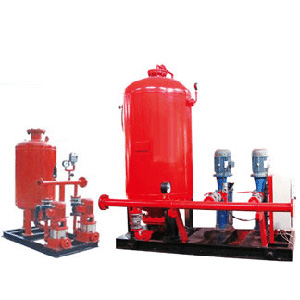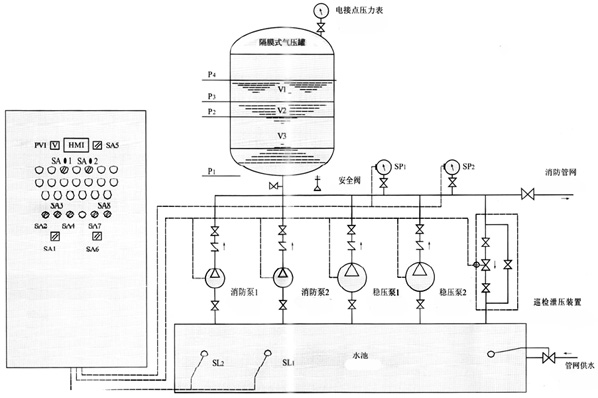﻿ 消防增压稳压设备-型号-价格 - 永嘉龙洋泵阀有限公司

### 供水设备••••# 消防增压稳压设备

1、变频供水设备的隔膜罐一次充气持久使用，能防止水质污染。
2、设备占地小投资省，自动运行。
3、故障保护，故障自动切换。
4、可与控制中心联网（可根据设计）
5、消防气压供水设备根据标准图集，98s205、GA30-92、GA30-2002等标准组织生产制造。

### 2消防增压稳压设备产品特点

1、变频供水设备的隔膜罐一次充气持久使用，能防止水质污染。
2、设备占地小投资省，自动运行。
3、故障保护，故障自动切换。
4、可与控制中心联网（可根据设计）
5、消防气压供水设备根据标准图集，98s205、GA30-92、GA30-2002等标准组织生产制造。

### 4消防增压稳压设备安装要求

1、供水设备产品安装前应仔细检查泵体流产内有无硬质物，以免运行时损坏叶轮和泵体。
2、安装时管路理量不允许加在泵上，以免使泵变形，影响正常运行。
3、拧紧地脚螺栓，以免起动时振动对泵性能产生影响。
4、供水设备在泵的进、出口管路上安装调节阀，在泵出口附近安装压力表，以控制泵在额定工况内运行，确保泵的正常使用。
5、排出管路如装逆止阀应装在闸阀的外面。
6、泵的安装方式分为硬性联接安装和柔性联接安装。

### 6消防增压稳压设备线路图### 7消防增压稳压设备选型对照表

 序号 增压稳压设备型号 消防压力(Mpa)P1 立式隔膜式气压罐 配用水泵 运行压力 (Mpa) 稳压水容积(L) 型号规格 工作压力比αb 消防储水容积(L) 型    号 标定容积 实际容积 1 ZW(L)-1-X-7 0.10 XQG800×0.6 0.60 300 319 25LG3-10×4 N=1.5kw P1=0.10  PS1=0.26 P1=0.23  PS2=0.31 54 2 ZW(L)-1-Z-10 0.16 XQG800×0.6 0.80 150 159 25LG3-10×4 N=1.5kw P1=0.16  PS1=0.26 P1=0.23  PS2=0.36 70 3 ZW(L)-1-X-10 0.16 XQG800×0.6 0.60 300 319 25LG3-10×5 N=1.5kw P1=0.16  PS1=0.36 P1=0.33  PS2=0.42 52 4 ZW(L)-1-X-13 0.22 XQG1000×0.6 0.76 300 329 25LG3-10×4 N=1.5kw P1=0.22  PS1=0.35 P1=0.32  PS2=0.40 97 5 ZW(L)-1-XZ-10 0.16 XQG1000×0.6 0.65 450 480 25LG3-10×4 N=1.5kw P1=0.16  PS1=0.33 P1=0.30  PS2=0.38 86 6 ZW(L)-Ⅰ-XZ-13 0.22 XQG1000×0.6 0.67 450 452 25LG3-10×5 N=1.5kw P1=0.22  PS1=0.41 P1=0.38  PS2=0.46 80 7 ZW(L)-Ⅱ-Z- A 0.22 -0.38 XQG800×0.6 0.80 150 159 25LG3-10×6 N=2.2kw P1=0.38  PS1=0.53 P1=0.50  PS2=0.60 61 8 B 0.38 -0.50 XQG800×1.0 0.80 150 159 25LG3-10×8 N=2.2kw P1=0.50  PS1=0.68 P1=0.65  PS2=0.75 51 9 C 0.50 -0.65 XQG1000×1.0 0.85 150 206 25LG3-10×9 N=2.2kw P1=0.65  PS1=0.81 P1=0.78  PS2=0.86 59 10 D 0.65 -0.85 XQG1000×1.6 0.85 150 206 25LG3-10×11 N=3.0kw P1=0.85  PS1=1.04 P1=1.02  PS2=1.10 57 11 E 0.85 -1.0 XQG1000×1.6 0.85 150 206 25LG3-10×13 N=4.0kw P1=1.00  PS1=1.21 P1=1.19  PS2=1.27 50 12 ZW(L)-Ⅱ-X- A 0.22-0.38 XQG800×0.6 0.78 300 302 25LG3-10×6 N=2.2kw P1=0.38  PS1=0.53 P1=0.50  PS2=0.60 72 13 B 0.38-0.50 XQG800×1.0 0.78 300 302 25LGW3-10×8 N=2.2kw P1=0.50  PS1=0.68 P1=0.65  PS2=0.75 61 14 C 0.50-0.65 XQG1000×1.0 0.78 300 302 25LG3-10×10 N=3.0kw P1=0.65  PS1=0.88 P1=0.86  PS2=0.93 51 15 D 0.65-0.85 XQG1200×1.6 0.85 300 355 25LG3-10×13 N=4.0kw P1=0.85  PS1=1.05 P1=1.02  PS2=1.10 82 16 E 0.85-1.0 XQG1200×1.6 0.85 300 355 25LG3-10×15 N=4.0kw P1=1.00  PS1=1.21 P1=1.19  PS2=1.26 73 17 ZW(L)-Ⅱ-XZ- A 0.22-0.38 XQG1200×0.6 0.80 450 474 25LG3-10×6 N=2.2kw P1=0.38  PS1=0.53 P1=0.50  PS2=0.60 133 18 B 0.38-0.50 XQG1200×1.0 0.80 450 474 25LG3-10×8 N=2.2kw P1=0.50  PS1=0.68 P1=0.65  PS2=0.75 110 19 C 0.50-0.65 XQG1200×1.0 0.80 450 474 25LG3-10×10 N=3.0kw P1=0.65  PS1=0.81 P1=0.78  PS2=0.86 90 20 D 0.65-0.85 XQG1200×1.6 0.80 450 474 25LG3-10×12 N=4.0kw P1=0.85  PS1=1.04 P1=1.02  PS2=1.10 73 21 E 0.85-1.0 XQG1200×1.6 0.80 450 474 25LG3-10×14 N=4.0kw P1=1.00  PS1=1.21 P1=1.19  PS2=1.27 64

• 来自【上海市】客户反馈

单位工作人员，消防稳压设备验货通过才来评价，CCCF认证，质量过关，消防资质齐全，比较专业的一家

• 来自【贵州省铜仁市】客户反馈

消防稳压设备外观漂亮，通电试了一下，声音能接受，还未安装通水。

• 来自【河南省郑州市】客户反馈

支架被物流扔坏了，老板二话不说寄了个新的过来，服务不错，就是物流那边消防泵厂家需要要交代下

• 来自【广西河池市】客户反馈

物流很给力 消防增压设备很快收到了。证书证件都很齐全 正规厂家，在店家的指导下安装好，试用了一下压力符合要求，杂音不大，很满意。

• 来自【福建省泉州市】客户反馈

买了套机组，发货速度非常快，已经安装调试了，声音也不大，正规消防设备厂家出的产品真是不错，这次领导们非常满意！